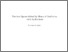Ricerca
Browse
Informazioni

Angrisani, Francesca (2021) Function spaces defined by means of oscillation, with application. [Tesi di dottorato]Preview
Text
Angrisani_Francesca_33.pdf

[error in script] [error in script]
Item Type: Tesi di dottorato
Lingua: English
Title: Function spaces defined by means of oscillation, with application
Creators:
CreatorsEmail
Angrisani, Francescafrancesca.angrisani@unina.it
Date: 13 April 2021
Number of Pages: 145
Institution: Università degli Studi di Napoli Federico II
Department: Matematica e Applicazioni "Renato Caccioppoli"
Dottorato: Matematica e applicazioni
Ciclo di dottorato: 33
Coordinatore del Corso di dottorato:
nomeemail
Tutor:
nomeemail
Moscariello, GiocondaUNSPECIFIED
Sbordone, CarloUNSPECIFIED
Date: 13 April 2021
Number of Pages: 145
Uncontrolled Keywords: oscillation, Lipschitz, Holder, Orlicz, Optimal Control, Regularity Theory, Calculus of Variation, (o,O)-pairs, BMO, VMO, BLO, VLO.
Settori scientifico-disciplinari del MIUR: Area 01 - Scienze matematiche e informatiche > MAT/05 - Analisi matematica
Date Deposited: 19 Apr 2021 18:04In the mathematical literature, a plethora of different meanings and formal definitions have been associated to the word "oscillation". In this text we will explore some of the function spaces defined by means of oscillation, in many different senses of the word, fitting into two modes: spaces in which oscillation is bounded and spaces in which oscillation is vanishing, i.e. arbitrarily small when measured on a sufficiently small set. This general framework will be made precise in a diversity of ways. The outline of the thesis is the following. In the first chapter a very large space of functions introduced by Brezis, Bourgain and Mironescu in 2015 and often denoted as $B$ is introduced. In Chapter 2 we explore the space of Lipschitz functions, in the most general setting of an arbitrary compact metric space and together with other function spaces from the family of Holder spaces. The last section of the Chapter 2 is devoted to some results obtained in the field of Optimal Control Theory. In Chapter $3$ we introduce Orlicz spaces for any choice of a Young function $\Psi$ and the closure of $L^\infty$ in $L^\Psi$, also known as the Morse space $M^\Psi$. We individuate a large subfamily of Orlicz spaces for which $(L^\Psi,M^\Psi)$ fits into a mathematical framework by K.M. Perfekt, deriving many functional properties of the couple.\\ In particular, in the last section of this chapter, we discuss some applications to the regularity of minima of some functionals in the calculus of variations. Chapters 4 and 5 are dedicated to the spaces $BMO$ and $VMO$ and their respective subcones $BLO$ and $VLO$, concluding the text with discussion of what are arguably the most natural "children" of the very large space $B$ discussed at the beginning.